Warwick Grace   of  Grace Research Network

11 April 2006

Published in Australian Meteorological Magazine June 2006

Abstract

An analytic model is presented for the broadband diffuse irradiance received at the earth’s surface through a cloudless homogeneous atmosphere containing  light absorbing agents and isotropic scattering agents. The model is extended to include a contribution from light re-scattered after  ground reflection. The model is validated, with some qualification, against (a) a Monte Carlo model based on the same physics,  (b) ground based observations at Adelaide and Alice Springs, and (c) two empirical models.

Motivation

Sophisticated and comprehensive spectral and broadband models of radiation requiring numerical solution (radiation transfer equation models) are well established in the study of direct and diffuse light (Mishchenko et al 2002, 2003; Bird and Riordan, 1984). At the other extreme are well-supported and simple empirical broadband models for diffuse irradiance in terms of the direct irradiance (Peterson and Dirmhirn 1981 and Campbell and Norman 1998, hereafter PD and CN respectively). The highly idealised broadband model developed in this paper provides an analytic expression for diffuse irradiance which is comparable in simplicity to the empirical models. This model also allows an a priori indication of the empirical constants in the empirical models. Compared to the more sophisticated numerical models, an analytic model of this nature provides an easy way to evaluate the sensitivity of diffuse irradiance to environmental factors of zenith angle, albedo, atmospheric transmittance, and the relative proportions of scattering and absorption agents in the atmosphere. As such it is suitable for illustrative or pedagogic purposes.

Introduction

At midday on a cloudless day,  about a quarter of the sun’s radiation is scattered or absorbed as it passes through the atmosphere. The radiation which reaches the earth's surface, coming from the direction of the sun, is called direct normal irradiance (or beam irradiance).Some of the scattered sunlight is scattered back into space and some of it reaches the surface of the earth. The scattered radiation reaching the earth's surface is called diffuse irradiance. Some radiation is reflected off the earth's surface and then re-scattered by the atmosphere back to the surface. This is also a component of the observed diffuse irradiance.Other components, ignored in this study, are the radiation reflected directly from clouds or topography. The total solar radiation on a horizontal surface is called global irradiance and is the sum of incident diffuse irradiance plus the direct normal irradiance projected onto the horizontal surface.

Diffuse irradiance in clear (cloud free) skies is typically 10% of the global irradiance. Air molecules scatter light while aerosols may act to either absorb and scatter light. Scattering by air molecules (Rayleigh scattering) is predominantly forward and backward of a photon’s pre-scattering direction; scattering by aerosols (Mie scattering) is predominantly transverse. Scattering and absorption are wavelength dependent and height dependent. Table 1 shows typical amounts of scattering and absorption due to the various atmospheric constituents. Water vapour is one of the largest causes in variability of  absorption and scattering.  Background information on light transmission through the atmosphere is available at the web sites of the Australian Bureau of Meteorology and other relevant international agencies. Comprehensive texts include  Kondratyev 1969,  Iqbal 1983, Goody and Yung 1989,  Bird and Riordan 1984, and Schwerdtfeger 1995.

 Constituent Percent absorbed Percent scattered Ozone 2 0 Water vapour 8 4 Dry air 2 7 Upper dust 2 3 Lower dust 0 0 Total ~  14 ~ 14

Table 1 Typical fractions of solar radiation absorbed or scattered in passing through cloudless atmosphere due to various constituents in the atmosphere (from Solar Radiation Monitoring Laboratory, University of Oregon, 2002)

In determining the radiation, knowledge of the variation of the solar constant and  the solar zenith angle (the angle of the sun to the vertical) are required. Useful formulas of sufficient accuracy are available from many sources (eg, Smithsonian Tables (List 1984), de Wit 2000)).  Half-hourly ground based observations of irradiance are available from the Bureau of Meteorology with the convenience that these are already tabulated against local solar time (ie, the equation of time, the longitude distance to the reference meridian for the local time zone, and any effect of daylight saving are already accounted for).  Figure 1 compares the daily variation of the extra-terrestrial, global, direct and diffuse irradiance for a cloud-free day at Adelaide Airport. Figure 2 shows in detail the variation of diffuse irradiance for the same day.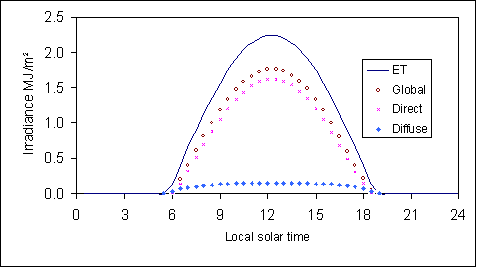Figure 1  Observed half-hourly exposures of global, direct and diffuse irradiance and calculated extra-terrestrial (ET) irradiance in MJ/m2 at Adelaide Airport on 17 October 2004.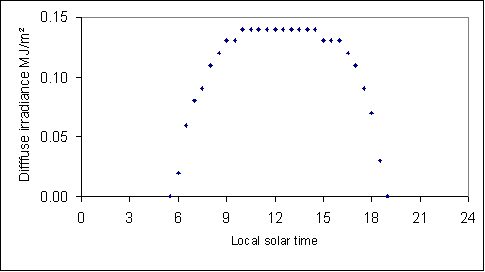.

Figure 2  Typical cloud-free diffuse irradiance. Half-hourly exposures in MJ/m2 observed at Adelaide Airport on 17 Oct 2004.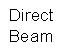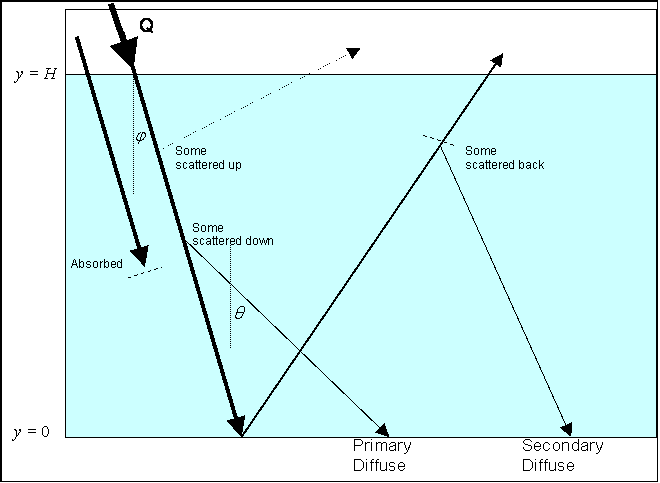Figure 3  Schematic of main features of the model. Incoming solar radiation, Q, at the top of the atmosphere may undergo absorption, scattering within the atmosphere or reflection from the ground. Once scattered or reflected, light may undergo subsequent scattering.

The Model

The model as depicted at Figure 3 assumes independence of wavelength and that all scattering is isotropic. Also assumed is an homogeneous atmosphere of finite depth, H, containing absorbing agents and scattering agents such that the extinction of the solar beam conforms to Beer’s Law (Eq 1). In the first instance, it is assumed that there is no ground reflection, ie, surface albedo is zero. The intensity  (q, the radiation energy per unit area on a surface normal to the beam) attenuates exponentially with distance traveled l such that

dq  =  - kq dl                                                                                                               (1)

where the constant k represents the concentration of intercepting agents: ka represents absorbers and ks the isotropic (multiple) scatterers so  k = ka + ks . In this paper, a scattering ratio, D, is defined as  ks / k.  Table 1 shows D is typically 0.5 and some inspection reveals that drier conditions imply D ~ 0.6 to 0.7 and moister conditions D ~ 0.3 to 0.4, all other things being equal.  Now let  Q represent the top of atmosphere irradiance (being the solar constant, Q ~1367 W/m2 ) and n  the zenith angle of the sun and set the vertical coordinate as y,  with y = 0 at the ground and y = H at the top of the atmosphere. Thus

dq    =  + k secn  q dy  .                                                                                             (1a)

Integrating Equation 1a and noting that q = Q at y = H, then

q    =   Q   e- k H secn   + k y secn                                                                                       (1b)

dq    =   Q k secn  e- k H secn   + k y secn dy                                                                        (1c)

Direct (beam) irradiance (normal to sun) at the surface     =   Q  e- k Hsecn                      (1d)

Direct irradiance on horizontal surface                                         =   Q cos n  e- kHsecn             (1e)

The product kH is known as the optical depth of the atmosphere, and the term e- kHsecn  is the transmittance or transmissivity, T, of the angled beam. Zenith transmittance, Tz, is the transmittance when n  is zero and is given by e- kH.  A typical value of Tz  for a clear day is 0.6 to 0.7 with values up to 0.8 for the clearest days (CN, McIlveen 1992).

The amount intercepted in the direct beam on a horizontal unit area (that is, integrating Equation 1c through a vertical column of height H ) is therefore   Q cosn (1 –  e- kH secn   ) and, of this amount, proportionately D is scattered and, through symmetry, ½ is downward scattered. Thus, the downward diffuse irradiance So is given by

So  =  ½ D Q cosn (1 –  e-kHsecn )                                                                                   (2)

Assume that this is distributed uniformly within the column (reasonable for clear sky) so that the downward scattered irradiance contribution dSo from a unit horizontal area element in the column of thickness dy is therefore

dSo =  ½ D Q cosn (1 – e-kHsecn ) (1/H)  dy                                                                    (3)

This amount may be envisaged as being emitted isotropically from a thin horizontal uniform slab. The flux density reaching the surface from such a source is known to attenuate as w where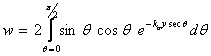.                                                                       (4)

and where 2 is the zenith angle for any scattered beam in question. Now it is apparent that  w  is a function not of 2 , but of the product ka and y, and it turns out that a good parameterization for w is given by(5)

where β . 1.66. And thus Equation 3 is modified by the attenuation factor, w, to become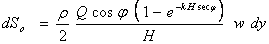.                                                               (6)

The derivation of the expression for w at Equation 4 is well established (eg Iqbal 1983, Schwerdtfeger 1995 and Coakley 2003) and is not provided here. However, intuitively one might expect that w would involve exponential attenuation in ka y.  Substituting Equation 5 into Equation 6 and integrating throughout the vertical column gives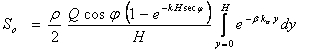(7)

and thus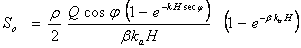.                                           (8)

Anticipating that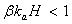(typical values are ~0.2), then Equation 8 may be approximated as

So  =   0.5D Q cosn (1 – e- kH secn)   (1 – 0.5βka H)                                                       (9)

Equation 9 is therefore an expression for zero-albedo diffuse irradiance. With increasing kaH, Equation 9 will tend to under-estimate. Replacing kaH with (1-D)kH gives

So  =   0.5D Q cosn (1 – e- kH secn)   (1 – 0.5β(1-D) k H) ,                                             (9a)

so that the diffuse irradiance is an analytic function of  zenith angle, n, of optical depth,  k H, and the scattering ratio, D.

Compared to photons scattered once only, those photons undergoing second and subsequent scattering have on average an increased path length. So the absorptive attenuation expected from Equation 5 will be an under-estimate of absorption.

Explicitly, the sources of error in the model as expressed by Equation 9 or 9a are as follows. The effect of multiple scattering means that the model over-estimates diffuse irradiance when D < 1. With increasing turbidity most of the initial absorption and scattering occurs in the upper part of the atmosphere and so the assumption of uniformity will break down  - leading to an over-estimate by the model. Thirdly, the simplifying approximation introduced at Equation 9 causes the model to under-estimate.

For convenience, allow So  to denote the primary diffuse irradiance. Now let S1  denote the secondary diffuse irradiance sourced from ground-reflected irradiance. Assume that the major component is from the reflected direct irradiance, neglecting any reflected So component. Assume that the albedo is A and that the ground reflection is Fresnel (where the angle of reflection equals the angle of incidence). Thus there is a surface source of upward irradiance  Q cos n A  e- k H secn.

With argument similar to that above, the interception (Int) of ground-reflected radiation is given by

Int  =  (1 – e -kH secn ) Q cosn A  e- kHsecn  .                                                        (10)

As before, the downward scattered contribution dS1  at the surface from a thin horizontal uniform scattering slab is:

dS1       =  ½ D w Int H-1 dy                                                                              (11)

dS1       =  ½ D w (1 – e -kH secn ) Q cosn A  e- kH secn  H-1 dy ,                            (11a)

and w is the atmospheric depletion of the beam that is scattered from the slab (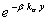). The final expression for S1 after integration and neglecting second order terms is:

S1 = 0.5D Q cosn (1 – 0.5β(1-D)k H) (1 – e -kH secn ) A  e- kH secn  .                    (12)

Since diffuse irradiance S ≈   S0  +  S1    (neglecting subsequent S2, S3, S4   terms)                         (13)

then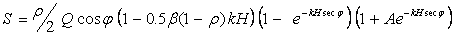.                 (14)

The neglect of the reflected So, S1, …, terms causes an under-estimating effect as ks  or A increases. This is the fourth source of error introduced by various assumptions and approximations. If Lambertian ground reflection (where the incident beam is reflected isotropically) were assumed then a slightly different expression for S results, namely,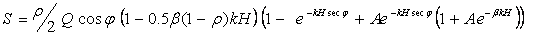.          (14a)

Equations14 and 14a clearly show that S increases with D or A, as intuitively expected. The effect of changes in n or kH are less obvious but are easily plotted for inspection.  An alternative perspective is that the analytic model as a function of n requires a knowledge of the three parameters Tz, D and A.

A comparison of the variants of the model with symmetrical reflection (Equation 14) and with isotropic surface reflection (represented by Equation 14a) is provided at Figure 4 with parameters Tz = 0.8, D = 0.5 and A = 0.25. The plots for each variant show that there is only a small difference between them. Other comparisons with a range of parameters, not shown, support this conclusion. The Fresnel variant for symmetrical reflection is used hereafter mainly because it is more amenable to comparison with the empirical models, and will be referred to as ‘the analytic model’.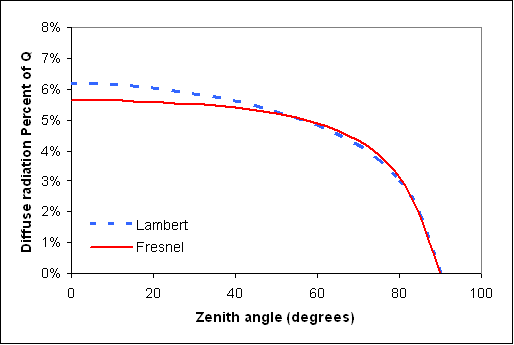Figure 4  Comparison of Fresnel and Lambert variants of the analytic model with parameters Tz = 0.8, D = 0.5 and A = 0.25.

Validation

Validation was performed in three ways: firstly, against output from a Monte Carlo model, having the same essential physics but not incorporating the several approximations used above, over a range of values of Tz, D, n  and A; secondly, against observational data of irradiance; and thirdly, against estimates from empirical models:

Monte Carlo model

A Monte Carlo model is easily constructed (less than a page of coding) and conceptually simple in that for the same model conditions, the atmosphere is comprised of 100 layers of thickness, h, where h = 0.01 H. Incoming solar radiation is represented by the  Q cosn   term above and in the form of N (~ 10 5 ) photons which are ‘fired’ into the top layer from above. Within any particular layer, each photon has a probability either of passing through uneventfully, or of being intercepted. The probabilities for either of these events are easily related to kh secn.  If a photon is intercepted it must then either be absorbed or scattered, with probabilities related to D . If it is scattered, then under the isotropic scattering condition, it scatters with equal probability at any solid angle. If it is neither scattered nor absorbed, then the photon passes into an adjacent layer where it is subject to the same process as before.  A photon reaching the ground is reflected symmetrically with probability equal to the albedo, otherwise it is absorbed. Each photon is tracked until either it is absorbed or it exits from the top of the atmosphere. Counts of photons corresponding to the irradiance components of direct, diffuse, absorbed, and that returned to space are easily made.

The Monte Carlo model was run for a comprehensive range of Tz , D, n  and A (this takes a few days on a modest PC). To obtain the diffuse irradiance, or other radiation components, for any specified combination of k, D, n  and A, then merely requires looking up a 4D table, interpolating as necessary.

For a range of Tz , D, n  and A, the results from the analytic model as expressed by  Equation 14 were compared to the Monte Carlo estimates of diffuse irradiance (see Figures 5a and 6a).  With low transmittance values (Tz < 0.33), the approximation introduced at Equation 9 is not used. Figure 5a shows comparative plots with zero albedo for a range of transmittance values. It is evident that the simple analytic model compares favourably with the Monte Carlo model at transmittance values greater than ~ 0.3 and with zero albedo. As noted by a reviewer, in the region of lower Tz with higher D ,  the deficiency of the model’s handling of multiple scattering becomes apparent; and this deficiency can be compensated for by introducing a correction factor. For the zero albedo case, a correction factor F0 can be applied as a multiplier to the right-hand side of Equation 8 where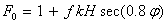(15)

and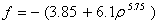(16)

Comparing the analytic model for zero albedo with this correction factor against the Monte Carlo estimates results in a very close agreement as shown at Figure 5b.

Figure 6a shows similar comparative plots using the analytic model (without any correction factor) with albedo of 0.25, 0.5 and 0.75 with Tz  limited to 0.6, 0.4 and 0.2. The comparisons are still quite good although the analytic model tends to strongly under-estimate with higher values of D and A  Thus, the analytic model with its approximations and assumptions fairly represents its simple physics. For completeness, a further correction factor, F, for non-zero albedo cases is provided here where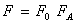(17)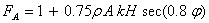(18)

As shown by the relevant comparison at Figure 6b, the corrected analytic model provides quite good agreement. As expected FA  reduces to 1 when A = 0. To be clear, the correction factor F is simply a mathematical construct arrived at by trial and error. It has the advantage of serving as a parameterization of the Monte Carlo model allowing a full range of possible scenarios to be easily explored. In general it would be difficult to develop a  parameterization with 4 variables without using the analytic model as the basis of a first guess estimate.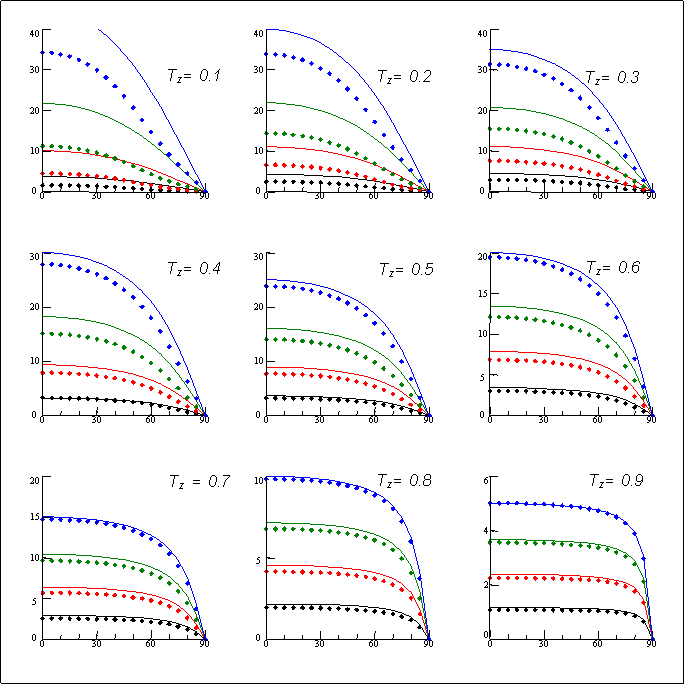Figure 5a  Comparison of analytic model (lines) with Monte Carlo model (diamonds) for a range of TZ , D and n  with albedo A = 0. Transmittance, TZ  = 0.1, 0.2,…0.9 for each panel reading left to right from top-left panel. The scattering ratio, D, varies within each panel from top to bottom as 1.0, 0.75, 0.50,  0.25. Zenith angle, n, is shown in degrees along the abscissa; the diffuse irradiance received at the surface as a percentage of the solar constant Q is shown on the coordinate axis. Note that the coordinate scale varies in order to better show relative differences. The analytic model compares favourably provided Tz > 0.3.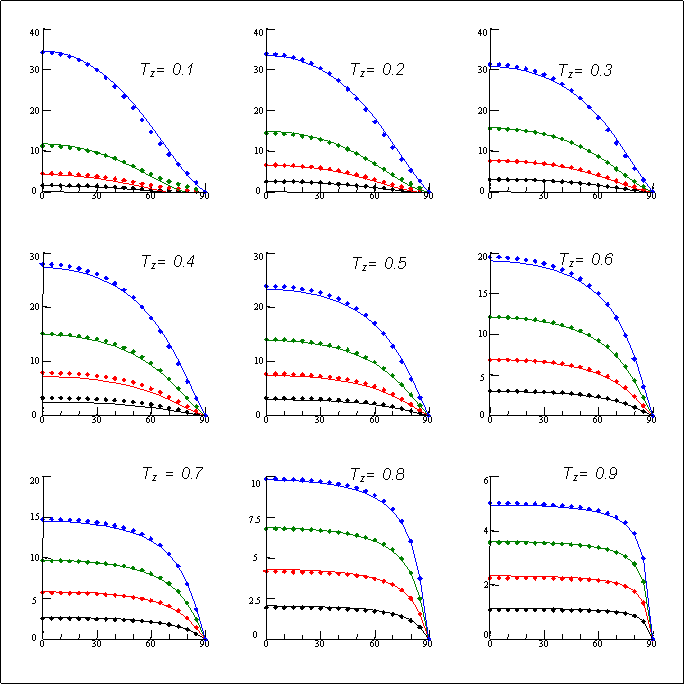Figure 5b  Comparison of corrected analytic model with Monte Carlo model as in Figure 5a.Figure 6a  Similar to Figure 5a but with albedo A = 0.25, 0.5 and 0.75 in rows from the top to bottom and  TZ  = 0.4, 0.6 and 0.8 in columns from left to right.  With lower transmittance, relatively more diffuse irradiance is received. With higher albedo, the irradiance increases and the increase is greater with larger D.  The analytic model still compares well but tends to strongly under-estimate for higher albedo as D  increases toward 1.0.